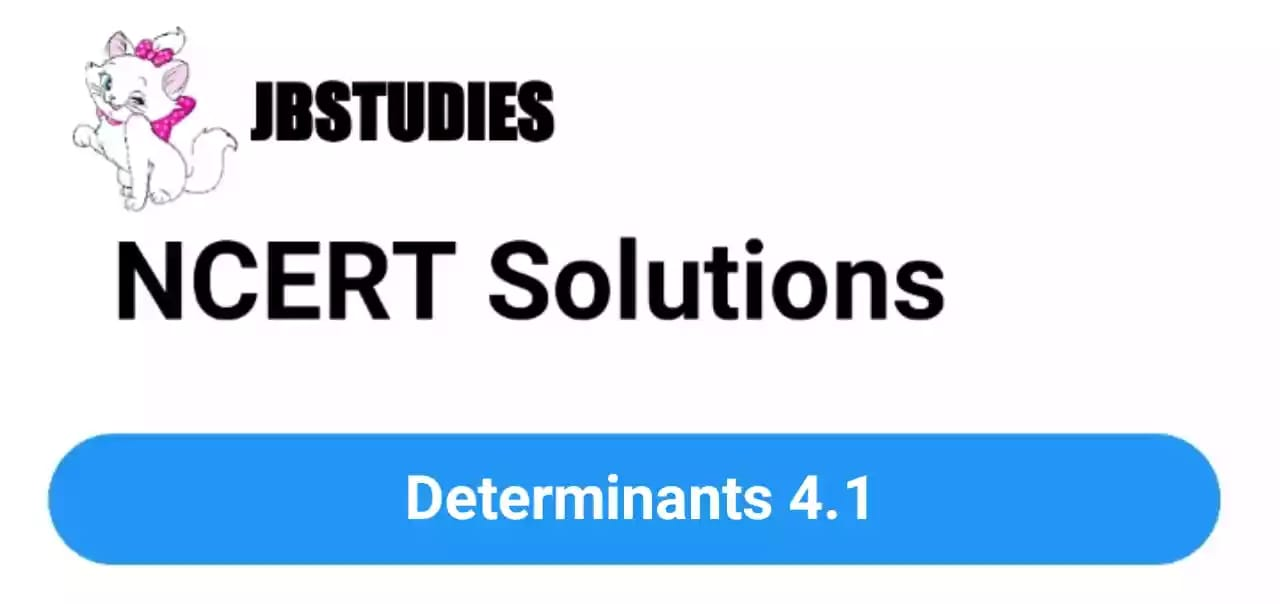# NCERT Solutions Class 12 maths Chapter-4 (Determinants)

NCERT Solutions Class 12 Maths from class 12th Students will get the answers of Chapter-4 (Determinants)Exercise 4.1 This chapter will help you to learn the basics and you should expect at least one question in your exam from this chapter.
We have given the answers of all the questions of  NCERT Board Mathematics Textbook in very easy language, which will be very easy for the students to understand and remember so that you can pass with good marks in your examination.Class 12 Mathematics

Chapter-4 (Determinants)

Questions and answers given in practice

Chapter-4 (Determinants)

### Exercise 4.1

Evaluate the determinants from the following Questions.

Question 1Solution:

The determinant of a 2 x 2 matrixHence,Question 2. (i)Solution:from trigonometric identities

(ii)Solution:Question 3. Ifshow thatSolution:

LHS=>

Matrix,Hence, determinant,RHS=>

Determinant,Now,Hence, proved, LHS = RHS

Question 4. Ifthen show that |Solution:

LHS=>

Matrix,Hence, determinant,RHS =>

Determinant,Now,Hence, proved, LHS = RHS

Question 5. Evaluate the determinants

(i)Solution:

Since the maximum number of zeroes are in the second row, we will expand the determinant along row 2.(ii)Solution:(iii)Solution:Note: This matrix is skew symmetric i.e.For every skew symmetric matrix of “odd dimension”, the determinant vanishes i.e. determinant is zero.

(iv)Solution:

Since the maximum number of zeroes are in the second row, we will expand the determinant along row 2.Question 6. Iffind |A|

Solution:Question 7. Find the values of x if

(i)Solution:

Solving determinants on both sides,(ii)Solution:

Solving determinants on both sidesQuestion 8. Ifthen x is equal to

(A) 6        (B)  ±6        (C) -6        (D) 0

Solution:

Solving determinants on both sidesHence, Option (B)